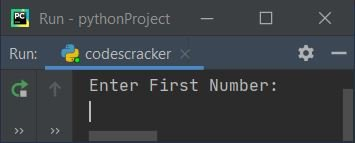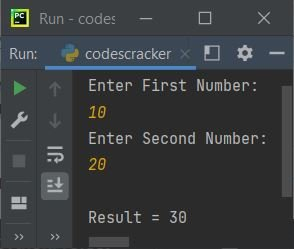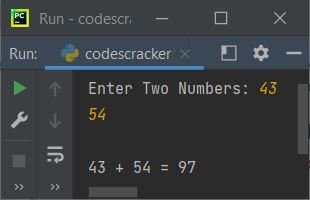# Python Program to Add Two Numbers

In this article, we've created many programs in Python to add two numbers using some popular approaches. Here are the list of programs to add two numbers in Python:

• Simple Program to Add Two Numbers
• Add Two Numbers using user-defined Function
• Using Recursion
• Using Class

## Add Two Numbers in Python

To add two numbers in python, you have to ask from user to enter any two numbers. Now find the sum of given two numbers using + operator and print the result on output as shown in the program given below.

The question is, write a Python program to add two numbers entered by user. Here is its answer:

```print("Enter First Number: ")
numOne = int(input())
print("Enter Second Number: ")
numTwo = int(input())
res = numOne+numTwo
print("\nResult =", res)```

This is the initial output, asking from the user to enter the first number:Now enter the first number say 10 and press `ENTER` key, then enter the second number say 20 and press `ENTER` key to add two given numbers and print the addition result on output as shown in the snapshot given below:#### Modified Version of Previous Program

This is the modified version of previous program. In this program, we've used float to allow user to enter floating-point value (including decimal point).

```print("Enter Two Numbers: ", end="")
nOne = float(input())
nTwo = float(input())
print(nOne, " + ", nTwo, " = ", nOne+nTwo)```

Here is its sample run with user input, 54.65 as first and 43.23 as second number:Note - The str() method is used to convert any type of value to a string type value. Because the concatenation using + operator can only performed with string type values.

Note - The end= is used to skip automatic printing of newline using print() statement.

## Add Two Numbers using Function

This program does the same job as of previous program. That is, to add two numbers, but using user-defined function named add(). This function takes two numbers as argument and returns its addition.

```def add(a, b):
return a+b

print("Enter Two Numbers: ", end="")
nOne = int(input())
nTwo = int(input())
print("\n" +str(nOne)+ " + " +str(nTwo)+ " = " +str(res))```

Here is its sample run with user input, 43 as first and 54 as second number:## Add Two Numbers using Recursion

This program uses recursive feature of function to do the same job. A recursive function is a function that calls itself from inside the function.

```def add(a, b):
if b==0:
return a
else:

print("Enter Two Numbers: ", end="")
nOne = int(input())
nTwo = int(input())

print("\n" +str(nOne)+ " + " +str(nTwo)+ " = " +str(res))```

This program produces same output as of previous program.

## Add Two Numbers using Class

This is the last program of this article. This program uses class and object, an object-oriented feature of Python language, to add two numbers.

```class CodesCracker:
return a+b

print("Enter Two Numbers: ", end="")
nOne = int(input())
nTwo = int(input())

cobj = CodesCracker()

print("\n" +str(nOne)+ " + " +str(nTwo)+ " = " +str(res))```

It also produces same output as of previous program. Using the statement, cobj = CodesCracker() all the properties of the class named CodesCracker gets assigned to this object. Now we've use this object (cobj) to access or call member function (add()) of the class CodesCracker

#### Same Program in Other Languages

Python Online Test

« Previous Program Next Program »